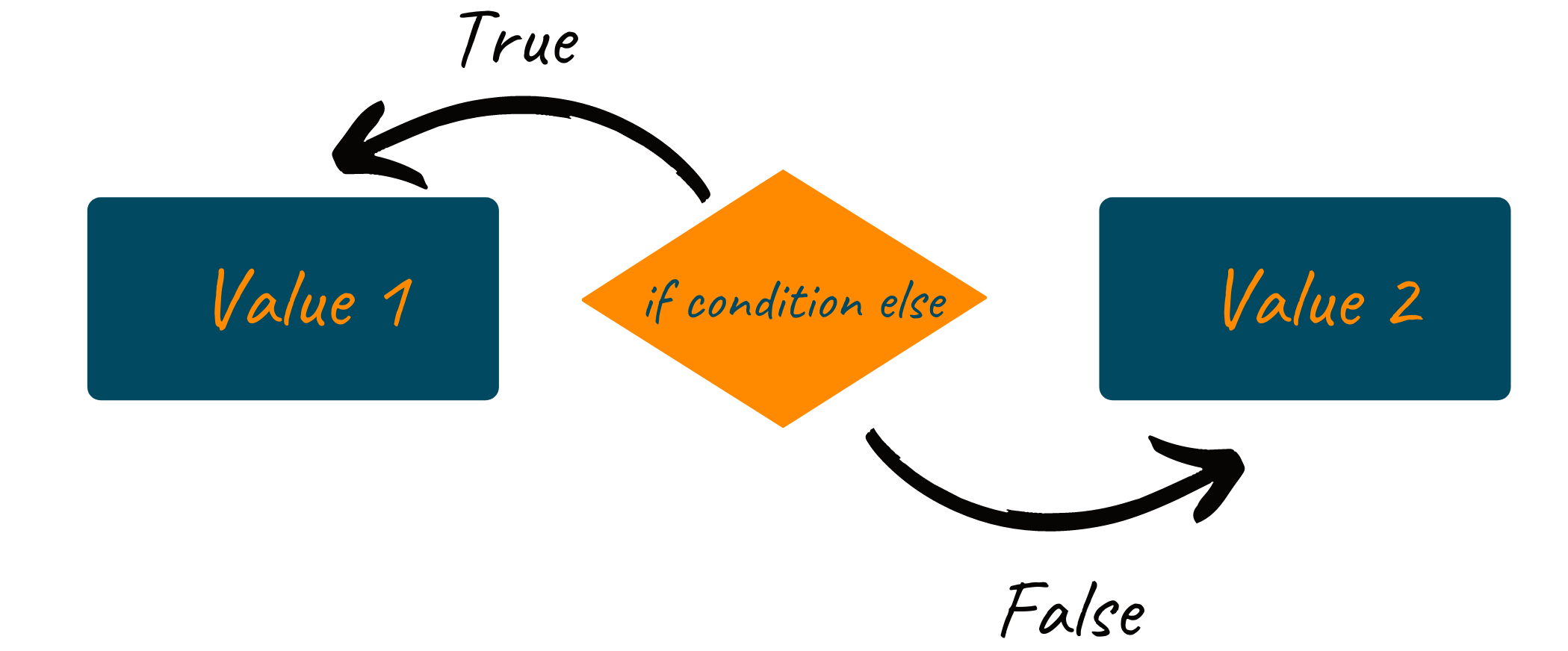# Python Ternary Operator With Examples

Writing clean, short, readable, and efficient code should always be the priority of any developer. Just for this reason, Python makes use of conditional statements and loops to avoid writing the same program and executing the same block of code over and over again until the condition is true. The ternary operator is one such shorter way of writing the conditional statement in Python. In this article, we will understand the python ternary operator and how it works, along with some examples and output.

## What is Ternary Operator in Python?

A conditional statement like the “if” statement helps you to control the flow of your program. The block of code inside the conditional statement gets executed when a particular condition is true. But while programming in Python, the conditional statement also requires enough lines of code to define the necessary condition and given code block. To solve this problem, the language offers a one-line code to test the condition and execute the code using the ternary operator.

Python ternary operator is the conditional expression to evaluate the statement while programming. It performs the action based on whether the given condition is true or false. Therefore, the ternary operator in Python is widely used as it is helpful to make your code compact and maintain all the necessary conditions.

As the name suggests, the python ternary operator consists of three operands:

• Condition: An expression to evaluate whether the condition is true or false. Condition is always Boolean type.
• True_value: The block of code to be executed if the boolean expression returns true.
• False_value: The block of code to be executed if the boolean expression returns false.### Syntax of the ternary operator in Python

```var = true_val if condition else false_val
```

For example:

```price = 40
discount = True if price >= 60 else False
print(discount)
```

Output

```False
```

## How to Use Ternary Operator in Python?

Below are some ways using which you can define the ternary operator in Python.

### 1) Using python tuples

You can use python tuples to specify the code to be executed if the condition returns True/False.

For example:

```import random
a = random.random()
b = random.random()
c = (b, a) [a>b]
print(c)
```

But here, you are confused whether it’s a or b, correct? Let us try this with another approach as shown below:

For example:

```import random
a = random.random()
b = random.random()
c = (f"b:{b}", f"a:{a}") [a>b]
print(c)
```

Output

```a:0.4429780625708817
```

Looking at the above code and its output, you’ll realize that the first argument in the tuple corresponds to "false_value," and the second corresponds to "true_value." It is because here, True=1 and False=0.

### 2) Using python dictionary

Just like tuples, you can also use this with a python dictionary using the same logic.

For example:

```import random
a = random.random()
b = random.random()
c = {False: f"b:{b}", True: f"a:{a}"}[a>b]
print(c)
```

Output

```b:0.7433253640750391
```

### 3) Using lambda

While programming in Python, the lambda function can also work as a ternary operator, as shown below:

For example:

```import random
a = random.random()
b = random.random()
c = (lambda: f"a:{a}", lambda: f"b:{b}")[a>b]()
print(c)
```

Output

```b:0.3130906547319653
```

### 4) Nested python ternary operator

Ever wondered what happens if we chain all these ternary operators? Let’s find out.

For example:

```import random
a = random.random()
b = random.random()
c = "Less than zero" if a<0 else "between 0 and 9" if a>=0 and a<=9 else "Greather than nine"
print(c)
```

Output

```between 0 and 9
```

In the above code, we check for the value of a. If it is below 0, we print “less than zero.” If it is between 0 and 9, we print “Between 0 and 9”, otherwise we print “Greater than 9”.

### 5) Shorthand ternary operator

In python programming, there is also the other version of the ternary operator named the shorthand ternary operator. This syntax was introduced in Python 2.5 and can be used in all versions above Python 2.5.

Syntax of shorthand ternary operator

```[expression] and [True_value] or [False_val]
```

In the above code, when the condition becomes true, the expression “true_value” is executed, whereas if the condition returns false, the expression “false_value” is executed.

For example:

```a = 5
b = 10
max = a > b and a or b

print(max)
```

Output

```10
```

The shorthand ternary operator is highly useful when you wish to quickly check the output of a function and execute a useful message if the output is empty.

## Conclusion

Python ternary operator is the most efficient and faster way to perform the simple conditional statement. It returns the specific block of code depending on whether the condition is true or false. As it is necessary to keep your code clean and readable, it is highly recommended to learn and understand the use of the python ternary operator as much as possible. To study more such programming topics, visit our blogs at Favtutor.

### FavTutor - 24x7 Live Coding Help from Expert Tutors!